## Content

### Sketching polynomial functions

Although polynomial graphs come in many shapes and sizes, they can be sketched once we find a few of their features.

#### Example

Sketch the graph of $$y=f(x)$$ where $$f(x) = 2x^3 - 6x^2 - 90x +350$$.

#### Solution

To find the $$x$$-intercepts, we solve $$f(x) = 2(x^3 - 3x^2 - 45x + 175) = 0$$. Trying factors of 175 we find that $$x=5$$ is a solution, so $$(x-5)$$ is a factor. We can then completely factorise $$f(x)$$ as $$2(x-5)^2(x+7)$$. So the $$x$$-intercepts are at $$x=5,-7$$. To find the $$y$$-intercept, we compute $$f(0) = 350$$.

To find the behaviour as $$x \to \pm \infty$$, note that $$f(x)$$ behaves like the leading term $$2x^3$$. So $$f(x) \to +\infty$$ as $$x \to +\infty$$, and $$f(x) \to -\infty$$ as $$x \to -\infty$$.

To find the stationary points, we solve $$f'(x) = 0$$. Differentiating gives

$f'(x) = 6x^2 - 12x - 90 = 6(x^2 - 2x - 15).$

Solving $$x^2 - 2x - 15 = 0$$ gives $$x=-3,5$$. Substituting these values into $$f$$ gives $$f(-3) = 512$$ and $$f(5) = 0$$. So the stationary points are $$(-3,512)$$ and $$(5,0)$$.

From this information, we can sketch the graph of $$y=f(x)$$.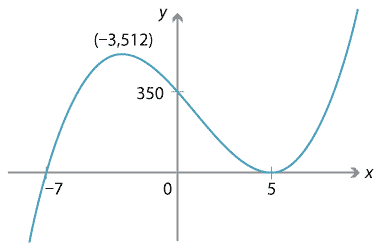Detailed description of diagram

Although it's unnecessary in the previous example, we could use a sign diagram to investigate stationary points. We choose values of $$x$$ before and after each stationary point, and consider whether $$f'(x) = 6(x+3)(x-5)$$ is positive or negative, as shown below.

 value of $$x$$ sign of $$f'(x)$$ slope of graph $$y=f(x)$$ −3 5 + 0 − 0 + $$\diagup$$ — $$\diagdown$$ — $$\diagup$$

From the sign diagram we see directly that $$x=-3$$ is a local maximum and $$x=5$$ is a local minimum. However in the example this is deduced from other information.

##### Exercise 10

Sketch the graph of $$y=x^3 - x$$.

In summary, the following information may be useful when sketching the graph of a polynomial $$y=f(x)$$:

• $$x$$-intercepts, obtained by solving $$f(x)=0$$
• $$y$$-intercept, obtained by evaluating $$f(0)$$
• behaviour as $$x \to \pm \infty$$, obtained by considering the leading term of $$f(x)$$
• stationary points, obtained by solving $$f'(x)=0$$
• sign diagram for $$f'(x)$$, obtained by substituting values into $$f'(x)$$.

It's not always necessary to calculate all of these; often, as in the previous example, a graph can be sketched with less information.

#### Repeated roots

When a polynomial has a factor $$(x-a)$$ to a power greater than 1, we say $$a$$ is a repeated root. If $$(x-a)^2$$ is a factor of $$f(x)$$, we say $$a$$ is a double root or a root of multiplicity 2. In general, if $$(x-a)^m$$ is a factor of $$f(x)$$, we say $$a$$ is a root of multiplicity $$m$$. A root of multiplicity 1 is often called a simple root.

Consider the polynomial $$f(x) = (x-a)^m$$, where $$a$$ is a real number and $$m$$ is a positive integer. Obviously $$f(x)$$ has a root at $$x=a$$ of multiplicity $$m$$. The following three graphs show $$y=f(x)$$ when $$m=1,2,3$$.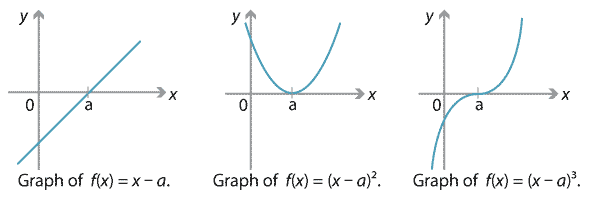Detailed description of diagrams

An important fact to note is that the sign of $$f(x)$$ changes at $$x=a$$ when $$m$$ is odd, and does not change when $$m$$ is even. (To see this, note that if $$x>a$$, then $$x-a$$ is positive and $$(x-a)^m$$ is also positive. If $$x<a$$, then $$x-a$$ is negative; if $$m$$ is even, then $$(x-a)^m$$ is positive, while if $$m$$ is odd, then $$(x-a)^m$$ is negative.)

This fact becomes relevant when we consider more general polynomials with repeated roots. Consider the polynomials $$f(x) = (x+2)^m (x-1)$$, where $$m$$ is a positive integer. Then $$f(x)$$ has a root at $$x=-2$$ of multiplicity $$m$$. The graphs for $$m=2,3,4$$ are shown below.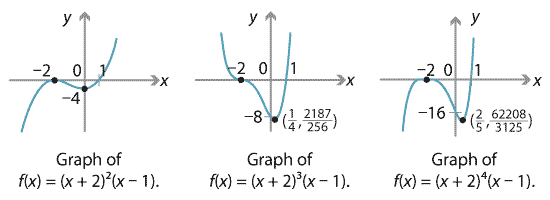Detailed description of diagrams

First consider the polynomial $$f(x) = (x+2)^2 (x-1)$$, which has a double root at $$x=-2$$. Then $$f(-2) = 0$$. Since the power 2 is even, the factor $$(x+2)^2$$ does not change sign at $$x=-2$$; if $$x$$ is slightly more or less than $$-2$$, then $$(x+2)^2$$ is positive. For $$x$$ close to $$-2$$, the factor $$x-1$$ is negative, and so $$f(x)$$ is negative for $$x$$ slightly more or less than $$-2$$. That is, $$f(x)$$ does not change sign at $$x=-2$$.

Next, consider $$f(x) = (x+2)^3 (x-1)$$, which has a triple root at $$x=-2$$. As 3 is odd, the factor $$(x+2)^3$$ does change sign at $$x=-2$$. For $$x$$ near $$-2$$, the factor $$x-1$$ is negative. So $$f(x)$$ changes sign at $$x=-2$$.

The above argument relies only on whether the multiplicity of the root $$x=-2$$ is even or odd. Using a similar argument, one can prove the following theorem.

###### Theorem

Let $$f(x)$$ be a polynomial with a root $$a$$ of multiplicity $$m$$. Then $$f(x)$$ changes sign at $$x=a$$ if and only if $$m$$ is odd.

If a polynomial $$f(x)$$ has a root at $$x=a$$ of even multiplicity $$m$$, then $$f(x)$$ does not change sign at $$x=a$$, and yet $$f(a)=0$$. Hence there must be a turning point and we obtain the next theorem.

###### Theorem

Let $$f(x)$$ be a polynomial with a root $$a$$ of even multiplicity. Then the graph of $$y=f(x)$$ has a turning point at $$x=a$$.

Additionally, we note from the above graphs that a root of multiplicity 2 or more always appears to be a stationary point. We can also prove this as a theorem.

###### Theorem

Let $$f(x)$$ be a polynomial with a root $$a$$ of multiplicity 2 or more. Then the graph of $${y=f(x)}$$ has a stationary point at $$x=a$$.

###### Proof
• Since $$a$$ has multiplicity at least 2, we know that $$(x-a)^2$$ is a factor of $$f(x)$$. (Maybe higher powers of $$(x-a)$$ divide into $$f(x)$$, but $$(x-a)^2$$ certainly does.)
• We can then write $$f(x) = (x-a)^2 g(x)$$ for some polynomial $$g(x)$$. Differentiate $$f(x)$$ using the chain and product rules to obtain
$f'(x) = 2(x-a) g(x) + (x-a)^2 g'(x) = (x-a) \big[ 2 g(x) + (x-a) g'(x) \big].$
• Thus $$f'(a) = 0$$ and so $$x=a$$ is a stationary point. $$\Box$$
##### Exercise 11

Sketch the graph of $$y = x^4 - x^2$$.

Screencast of exercise 11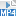#### Transformation methods

We've seen from the module Functions II that, if we take the graph of $$y=f(x)$$ and dilate or translate it in the $$x$$ and $$y$$ directions, or reflect in the axes, then the result is a graph of $$y=af(b(x-c))+d$$, for some real numbers $$a,b,c,d$$.

We can sometimes use this idea in reverse, as in the following example. It's easier than using the standard method.

#### Example

Sketch the graph of $$y=2 (x-1)^4 + 7$$.

#### Solution

We see that the graph is obtained from $$y=x^4$$ by successively:

• dilating by a factor of 2 from the $$x$$-axis in the $$y$$-direction
• translating 1 to the right
• translating 7 upwards.

Since we know the graph of $$y=x^4$$, the graph is easily obtained.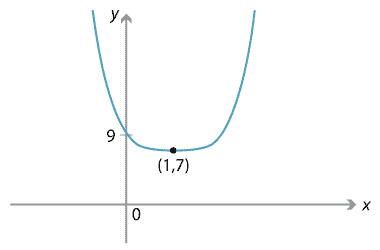Detailed description of diagrams

##### Exercise 12

Use the graph of $$y=x^5$$ to sketch the graph of $$y=-4 (x-3)^5 -2$$.

Next page - Content - Polynomials from graphs